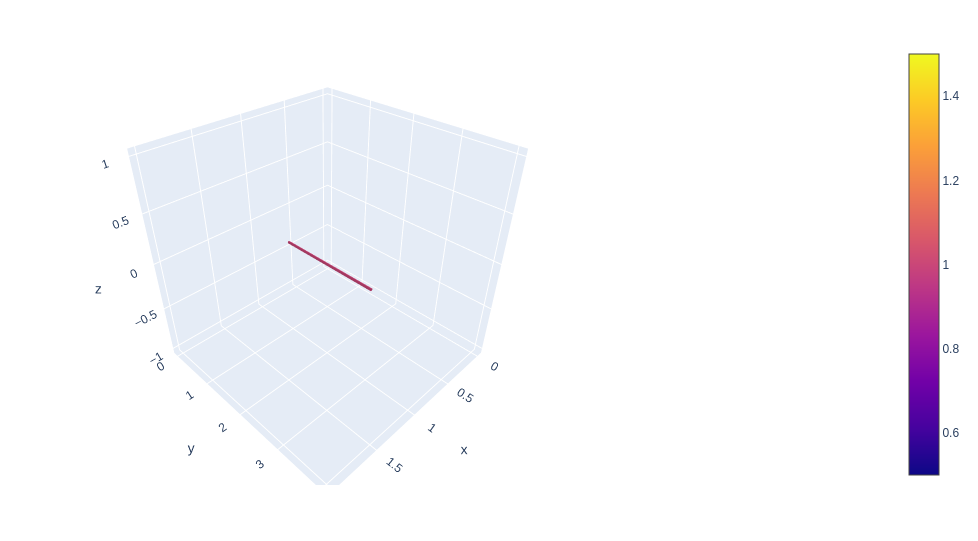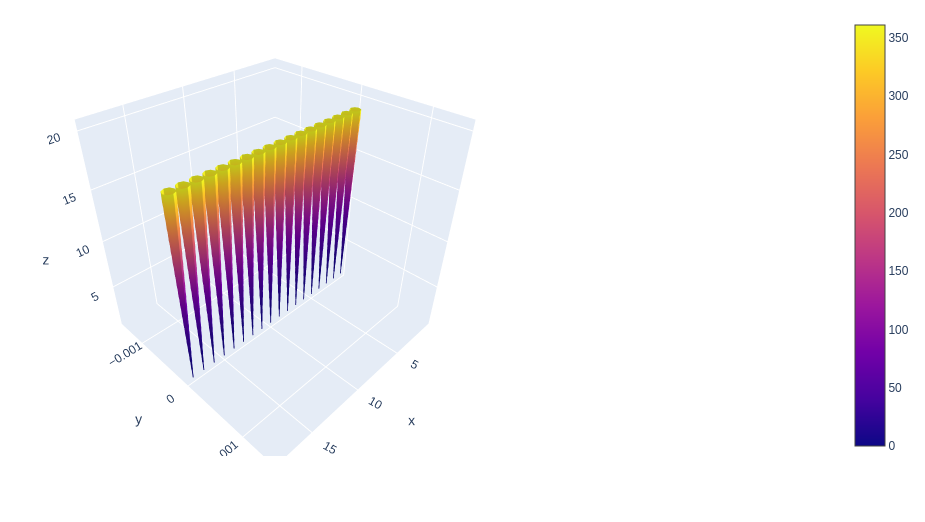GeeksforGeeks App
Open AppBrowser
Continue

# 3D Streamtube Plots using Plotly in Python

Plotly is a Python library that is used to design graphs, especially interactive graphs. It can plot various graphs and charts like histogram, barplot, boxplot, spreadplot, and many more. It is mainly used in data analysis as well as financial analysis. plotly is an interactive visualization library.

## 3D Streamtube Plots

In plotly, the streamtube plot, parameter includes X, Y, and Z, which set the coordinates with vector fields. U, V, and W which set X, Y, and Z component of vector fields. A streamtube is a tubular region surrounded by streamlines that form a closed loop.

Syntax: plotly.graph_objects.Streamtube(arg=None, hoverinfo=None, showscale=None, u=None, v=None, w=None, x=None, y=None, z=None, **kwargs)

Parameters:

arg: dict of properties compatible with this constructor or an instance of plotly.graph_objects.Streamtube

hoverinfo: Determines which trace information appear on hover. If none or skip are set, no information is displayed upon hovering. But, if none is set, click and hover events are still fired.

showscale: Determines whether or not a colorbar is displayed for this trace.

u: Sets the x components of the vector field.

v: Sets the y components of the vector field.

w: Sets the z components of the vector field.

x: Sets the x coordinates of the vector field.

y: Sets the y coordinates of the vector field.

z: Sets the z coordinates of the vector field.

Example:

## Python3

 `import` `plotly.graph_objects as go`` ` `fig ``=` `go.Figure(data``=``go.Streamtube(x``=``[``1``, ``1``, ``1``], y``=``[``1``, ``1``, ``2``],``                                   ``z``=``[``0``, ``0``, ``0``], u``=``[``0``, ``0``, ``0``], ``                                   ``v``=``[``1``, ``1``, ``1``], w``=``[``0``, ``0``, ``0``]))``fig.show()`

Output:## Changing Tube diameter

The diameter of tubes can be determined by local divergence of the vector field. The norms are proportionate but the direction of the vector is different and it result in different divergence field.

Example:

## Python3

 `import` `plotly.graph_objects as go``import` `numpy as np`` ` `x, y, z ``=` `np.mgrid[``0``:``20``, ``0``:``20``, ``0``:``20``]``x ``=` `x.flatten()``y ``=` `y.flatten()``z ``=` `z.flatten()`` ` `u ``=` `np.zeros_like(x)``v ``=` `np.zeros_like(y)``w ``=` `z``*``*``2`` ` `fig ``=` `go.Figure(data``=``go.Streamtube(x``=``x, y``=``y, z``=``z, u``=``u, v``=``v, w``=``w))``fig.show()`

Output:My Personal Notes arrow_drop_up